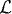# EXTENSION OF THE ITÔ INTEGRAL

In this chapter, we define the Itô integral for stochastic processes in a larger space.

# 33.1 Basic Concepts and Facts

Definition 33.1 (Stochastic Process Space. Letbe a filtration under consideration. The spacead(Ω, L2[a, b]) is defined to be the space of all stochastic processes f(t, ω), t[a, b], ωΩ, satisfying the following conditions:

(a) f(t) is adapted to thet.
(b)a.s.

Definition 33.2 (Extension of the Itô Integral). Let {t : atb} be a filtration. Let {Bt : atb} be a Brownian motion satisfying the following conditions:

(a) For each t, Bt is -measurable.
(b) For any st, the random variable BtBs is independent of s.

Get Measure, Probability, and Mathematical Finance: A Problem-Oriented Approach now with the O’Reilly learning platform.

O’Reilly members experience live online training, plus books, videos, and digital content from nearly 200 publishers.